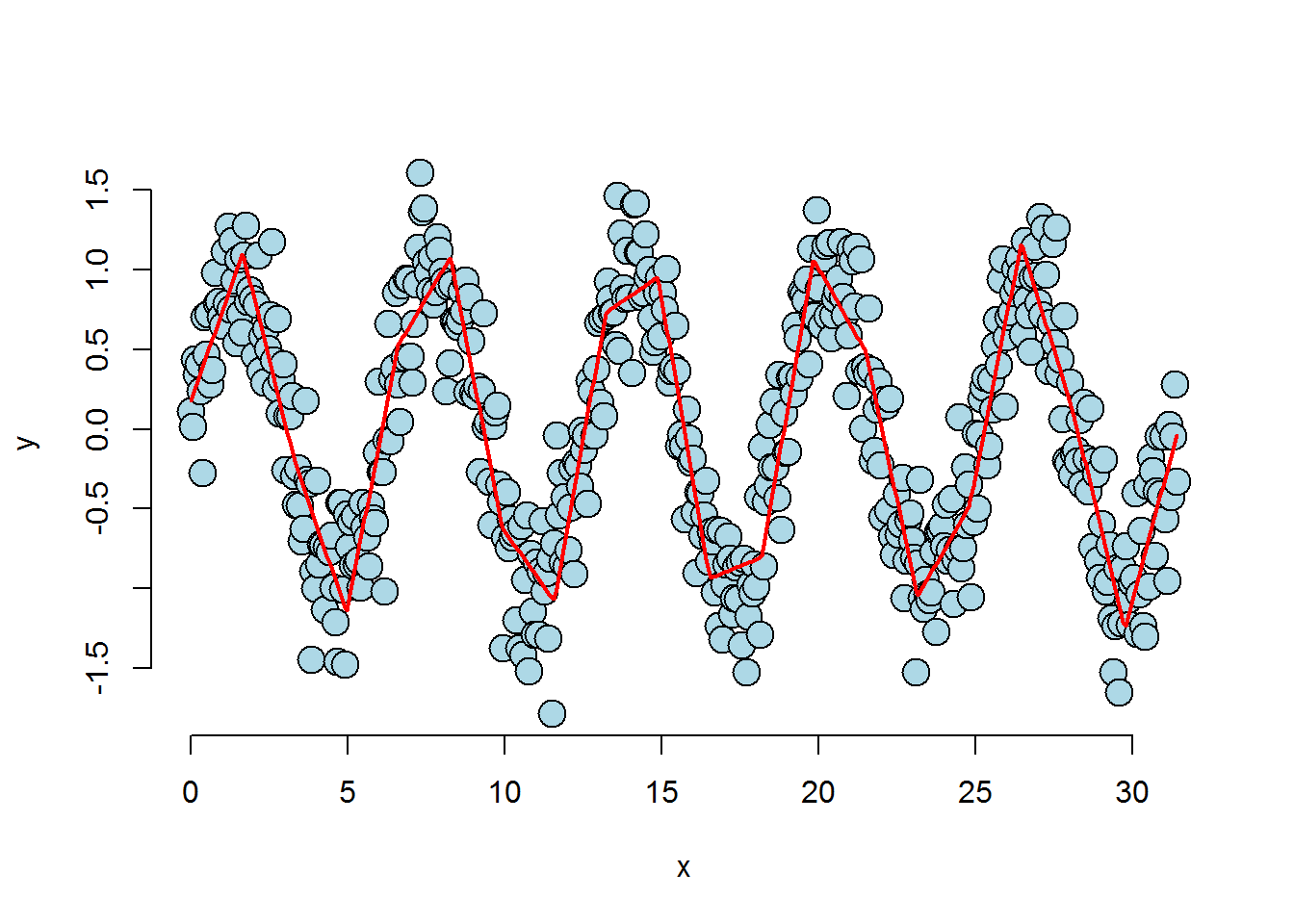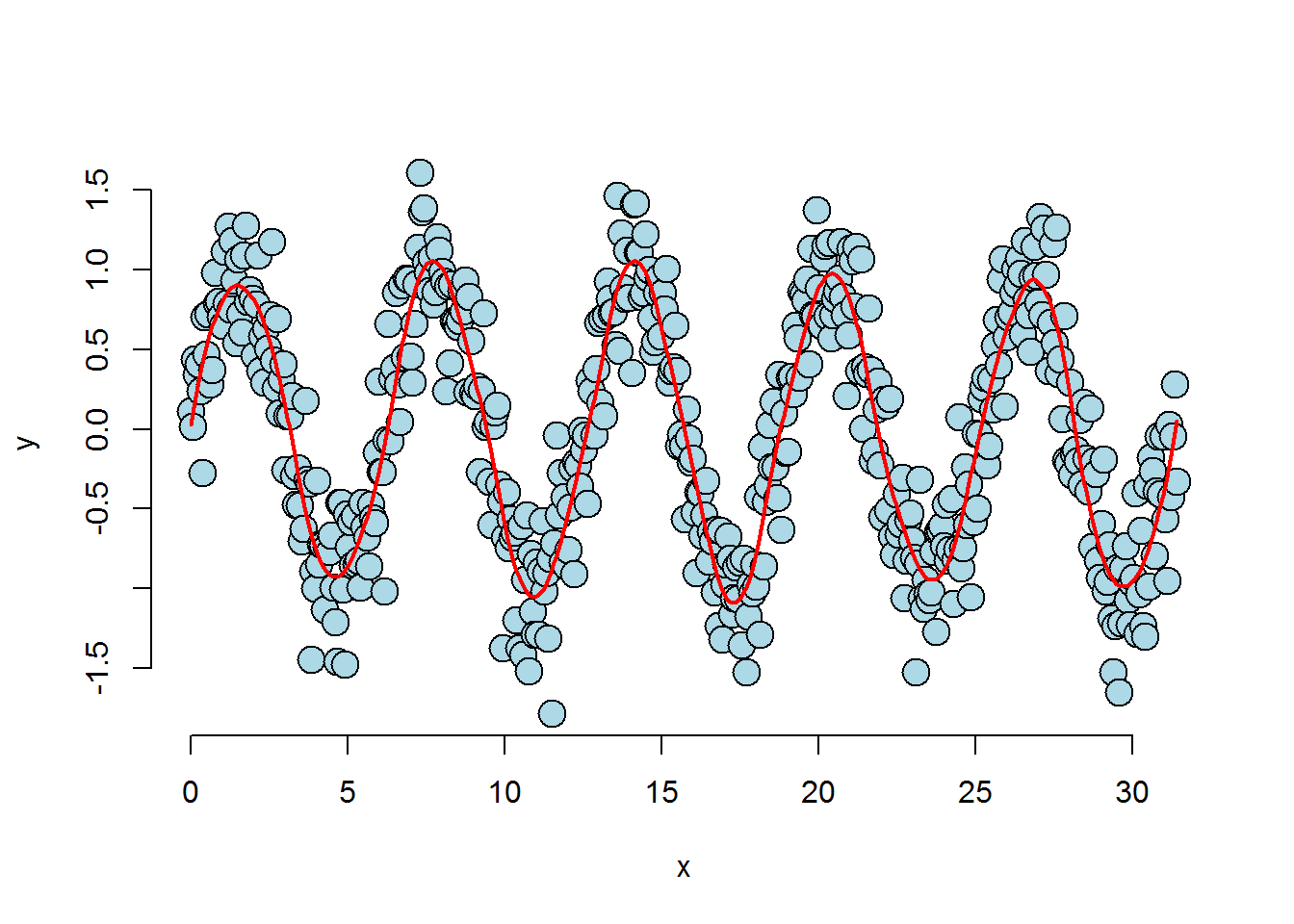# Fitting a function

If you see an error in the article, please comment or drop me an email.

# How to fit functions using linear models

$Y_i = \beta_0 + \beta_1 X_i + \sum_{k=1}^d (x_i – \xi_k)_+ \gamma_k + \epsilon_{i}$

## Simulated example

Source: https://github.com/DataScienceSpecialization/courses

1. Separate the n values into k+1 spans. (k standing for knots)

2. Create a basis: a collection of regressors or call it a set of values allowing to predict the regression line within each span. This can be done with spline terms: variables with null value below knot #n and distance value (x from the knot) above knot #n. Note that there are many types of spline bases.

3. These spline terms, combined with the original x values into one dataset, allow to predict the regression line.

#create x and y variables
n <- 500; k <- 20; x <- seq(0, 10 * pi, length = n); y <- sin(x) + rnorm(n, sd = .3)
#create knot terms to split the x values into (here) 20 spans, allowing to draw the regression line along the values
knots <- seq(0, 10* pi, length = k);
#create splineterms which add precision to the prediction model further down after each knot. There will be one additional variable for each knot/span.
splineTerms <- sapply(knots, function(knot) (x > knot) * (x - knot))
#create dataset allowing to predict y values
xMat <- cbind(1, x, splineTerms)
#predict y values based on model fuelled by splineterms
yhat <- predict(lm(y ~ xMat - 1))

#plot "original" values
plot(x, y, frame = FALSE, pch = 21, bg = "lightblue", cex = 2)
lines(x, yhat, col = "red", lwd = 2)### Smooth the regression line

Smooth the regression line by squaring the distance between x and the knots:

splineTerms <- sapply(knots, function(knot) (x > knot) * (x - knot)^2) #here
xMat <- cbind(1, x, x^2, splineTerms) #and here
yhat <- predict(lm(y ~ xMat - 1))

#plot "original" values and add regression line
plot(x, y, frame = FALSE, pch = 21, bg = "lightblue", cex = 2)
lines(x, yhat, col = "red", lwd = 2)